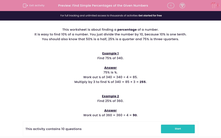# Find Simple Percentages of the Given Numbers

In this worksheet, students find simple, well-known percentages of the given numbers.Key stage:  KS 2

Curriculum topic:   Maths and Numerical Reasoning

Curriculum subtopic:   Percentages

Difficulty level:#### Worksheet Overview

This worksheet is about finding a percentage of a number.

It is easy to find 10% of a number. You just divide the number by 10, because 10% is one tenth.

You should also know that 50% is a half, 25% is a quarter and 75% is three-quarters.

Example 1

Find 75% of 340.

75% is ¾.

Work out ¼ of 340 = 340 ÷ 4 = 85.

Multiply by 3 to find ¾ of 340 = 85 × 3 = 255.

Example 2

Find 25% of 360.